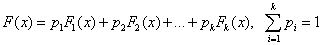ID 772987
Date 12/04/2020
Public

## Mixture of Distributions

You can split the initial distribution into several simpler distributions:In this case, random numbers for each of the distributions Fi(x) are easy to generate. An appropriate algorithm may be as follows:

1. Generate a random number i with the probability pi.

2. Generate a random number y (independent of i) with the distribution Fi(x).

3. Accept y as a random number x with the distribution F(x).

This technique is common in the acceptance/rejection method, when for the whole range of acceptable x values a density g(x), which would approximate function f(x) well enough, is hard to find. In this case, the range is divided into sections so that g(x) looks relatively simple in each of the sub-ranges.

NOTE:

Since quasi-random sequences are non-random, you should be careful when using quasi-random basic generators with the mixture methods.